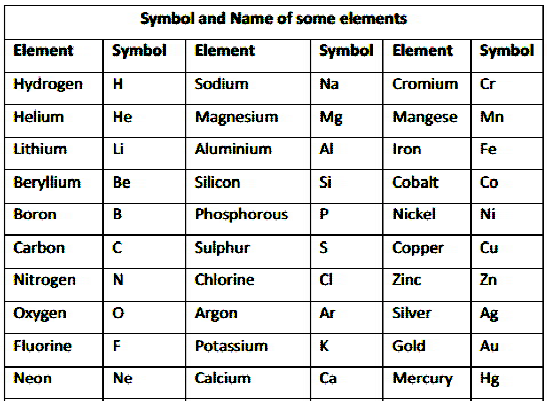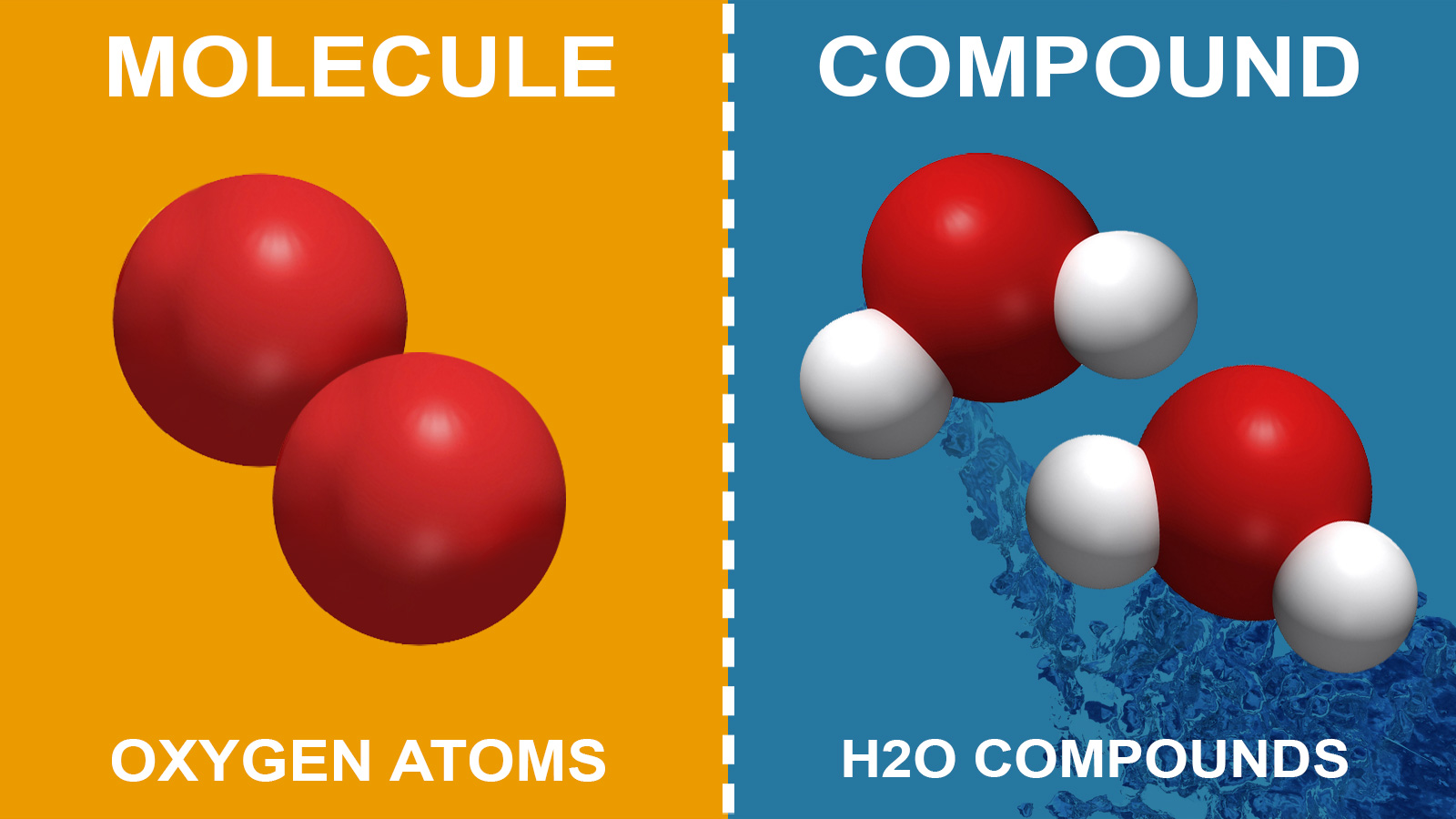# CBSE Class 9 Science Chapter 3 Revision Notes

Chapter 3:Atoms And Molecules Revision Notes

Atoms and molecules are responsible for the formation of everything from microscopic sand particles to massive black holes. Everything we see around us is made up of atoms, which are the most fundamental unit of matter. It is incredibly minute, measuring between 0.1 to 0.5 nanometers in diameter.

## LAWS OF CHEMICAL COMBINATIONS

1. Chemical Reactions

• Two or more molecules combine to generate new compounds in a chemical reaction, and these molecules are referred to as reactants, while the newly produced compounds are referred to as products.
• A chemical change must occur in a chemical reaction, which is commonly observed with physical changes such as precipitation, heat generation, colour change, and so on.

Law of conservation of mass

• Matter cannot be generated or destroyed in a chemical process, according to the rule of conservation of mass. It hasn’t changed.
• The mass of the reactants and the mass of the products will be equal.

Law of constant proportions

• The law of definite proportions states that a pure chemical compound comprises the same components mixed in a constant proportion by mass.
• If we take water from a river or an ocean, for example, both contain the same percentage of oxygen and hydrogen.

Limitation of “Law of definite proportion”

When the compound is made up of distinct isotopes of the combining elements, this rule does not apply.

2. Atomic Theory by John Dalton

Dalton rationalized the different rules of chemical combination that existed at the time using his theory. He did, however, think that the simplest two-element composite must be binary.

3. Atoms, Molecules, Ions & Chemical Formula

Atom

• An atom is the smallest unit of an element capable of participating in a chemical process. It may or may not be free to exist.
• Each atom of an element represents all of the element’s attributes.

Molecule

• The tiniest particle of matter (element or compound) capable of existing in its natural condition.
• A substance’s qualities are the characteristics of its molecules.

MOLECULES OF ELEMENT:

An element’s molecules are made up of the same sort of atoms.

MOLECULES OF COMPOUND:

Compound molecules are formed when atoms of various elements combine in certain quantities. (molecules with heteroatomic atoms)

ATOMICITY:

• The atomicity of a material (element or compound) refers to the number of atoms contained in a molecule.
• Hydrogen, nitrogen, oxygen, chlorine, iodine, and bromine, for example, all have two atoms in their molecules. Therefore, the atomicity of Hydrogen, nitrogen, oxygen, chlorine, iodine, and bromine is 2.

SYMBOLS

• The acronym for an element is usually the initial letter in capital letters of the element’s English name.

Oxygen (O)

Nitrogen (N)

• When the names of two or more elements begin with the same initial letter, the element is symbolised by the initial letter followed by the letter that appears later in the name.

Barium (Ba)

Bismuth (Bi)Source:

## ATOMIC MASS

Atomic mass and atomic mass unit

The average mass of an atom, or a set of atoms, is the sum of the masses of the electrons, neutrons, and protons.

The atomic mass is the mass of an atomic particle.

This is often stated in terms of a unified atomic mass unit, as per the international agreement (AMU).

1/12 of the mass of a carbon-12 atom in its ground state is the best definition.

Molecular mass

The total of the masses of the elements contained in a molecule is the molecule’s molecular mass.

The atomic mass of an element is multiplied by the number of atoms in the molecule, and then the masses of all the elements in the molecule are added.

Mole Concept

• A mole is the quantity of entities existing in a substance, such as atoms, molecules, and ions. A mole is 6.0221023 molecules of any material.
• One of the most practical methods of describing the number of reactants and products in a reaction is to use the mole idea.
• Avogadro’s number has a value of about 6.0221023. Avogadro’s number is a formula that calculates the number of particles in one mole (or mol) of a material. It’s possible that these particles are electrons, molecules, or atoms.

## WRITING CHEMICAL FORMULAE

CompoundsSource:

• When two or more elements join chemically in a defined mass ratio, the result is known as a compound.
• Compounds are substances made up of two or more different types of atoms in a certain ratio.

Ions

• An ion is an atom or molecule with a net positive or negative charge due to the gain or loss of one or more of its valence electrons.
• An anion is a negatively charged particle, whereas a cation is a positively charged particle.

Chemical formula for ionic compounds

• Ionic compounds are chemical compounds in which ions are bound together by ionic bonds, which are unique bonds that hold ions together.
• The positive and negative charges in an ionic compound are always equal.
• Consider the following scenario: The ionic bond in calcium chloride is formed by oppositely charged calcium and chloride ions.
• The electron configuration of the calcium atom is changed to that of the closest noble gas after it loses two electrons (Ar). It earns a net charge of +2 by doing so.

Source:

]]>